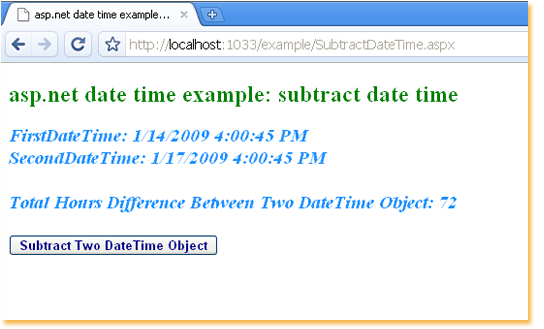### c# - How to subtract two DateTimes

Subtract two DateTimes
The following asp.net c# example code demonstrate us how can we subtract two DateTime objects programmatically at run time in an asp.net application. .Net framework's DateTime.Subtract() method allow us to subtract the specified time or duration from this instance. DateTime.Subtract() method is overloaded, those are DateTime.Subtract(DateTime) and DateTime.Subtract(TimeSpan).

DateTime.Subtract(DateTime) overloaded method subtract the specified date and time from the instance. We need to pass a DateTime object to this method as parameter. This method return a System.TimeSpan type value. The return TimeSpan object represent a time interval that is equal to date and time of instance minus date and time of parameter.

DateTime.Subtract(TimeSpan) overloaded method subtract the specified duration from this instance. This method required to pass a TimeSpan object as parameter. The TimeSpan represent the time interval to subtract. This overloaded method return a System.DateTime object which is equal to date and time of instance minus time interval of parameter.

After getting the return TimeSpan value from DateTime.Subtract(DateTime) method, we can convert the TimeSpan to total days, hours, minutes, seconds etc. So we can get total days, hours, minutes difference between two date time objects.
SubtractDateTime.aspx
``````
<%@ Page Language="C#" %>

<!DOCTYPE html>

<script runat="server">
protected void Button1_Click(object sender, System.EventArgs e) {
DateTime firstDateTime = DateTime.Now;
TimeSpan dateTimeDifference;
dateTimeDifference = secondDateTime.Subtract(firstDateTime);

double totalHours = dateTimeDifference.TotalHours;

Label1.Text = "FirstDateTime: " + firstDateTime.ToString();
Label1.Text += "<br />SecondDateTime: " + secondDateTime.ToString();
Label1.Text += "<br /><br />Total Hours Difference Between Two DateTime Object: " + totalHours;
}
</script>

<html xmlns="http://www.w3.org/1999/xhtml">
<title>asp.net date time example: how to subtract between two date time object</title>
<body>
<form id="form1" runat="server">
<div>
<h2 style="color:Green">asp.net date time example: subtract date time</h2>
<asp:Label
ID="Label1"
runat="server"
Font-Size="Larger"
Font-Italic="true"
Font-Bold="true"
ForeColor="DodgerBlue"
>
</asp:Label>
<br /><br />
<asp:Button
ID="Button1"
runat="server"
Font-Bold="true"
ForeColor="DarkBlue"
OnClick="Button1_Click"
Text="Subtract Two DateTime Object"
/>
</div>
</form>
</body>
</html>
``````More c# examples